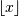# numpy.floor¶

numpy.floor(x[, out]) = <ufunc 'floor'>

Return the floor of the input, element-wise.

The floor of the scalar x is the largest integer i, such that i <= x. It is often denoted as.

Parameters: x : array_like Input data. y : ndarray or scalar The floor of each element in x.

Notes

Some spreadsheet programs calculate the “floor-towards-zero”, in other words floor(-2.5) == -2. NumPy instead uses the definition of floor where floor(-2.5) == -3.

Examples

```>>> a = np.array([-1.7, -1.5, -0.2, 0.2, 1.5, 1.7, 2.0])
>>> np.floor(a)
array([-2., -2., -1.,  0.,  1.,  1.,  2.])
```

numpy.fix

numpy.ceil